Tags Math

A linear combination of a and b is some integer of the form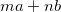, where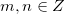.

There's a very interesting theorem that gives a useful connection between linear combinations and the GCD of a and b, called Bézout's identity:

Bézout's identity: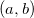(the GCD of a and b) is the smallest positive linear combination of non-zero a and b.

Both Bézout's identity and its corollary I show below are very useful tools in elementary number theory, being used for the proofs of many of the most fundamental theorems. Let's see why it's true.

(I) Intuition: First I'd like to explain this (surprising at first sight) theorem intuitively. By defintion, any common divisor of a and b will dividefor all. In particular,also divides any.

Now, assume we've found some small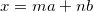which isn't the GCD. But we've just said thatdivides all linear combinations, so it also divides x. Therefore, x can not be smaller than the GCD. In other words, the smallest positive linear combination can only beitself.

Corollary: An integer is a linear combination of a and b IFF it is a multiple of their GCD.

To prove Bézout's identity more formally, and along the way to see why the corollary is also true, let's first prove the following:

(III) Let I be a nonempty set of integers that is closed under addition and subtraction, and contains at least one non-zero integer. Then there exists a smallest positive element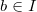, and I consists of all multiples of b (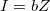).

Proof: I contains at least one non-zero integer. Then it definitely contains at least one positive integer, because it is closed under addition and subtraction. Assume we have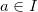for some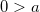. Therefore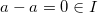and then also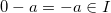. Thus we have positive integers in I. According to the well-ordering principle, I has a smallest positive element which we'll call b.

Now we'll want to show that. As usual, to prove equalities of sets, it will be shown that they contain one another.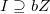is obvious - since I contains b and is closed under addition and subtraction, it contains all the multiples of b.

To prove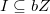we'll demonstrate that any element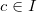is a multiple of b. Using the division algorithm we write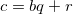for some integers q and 0 <= r < b. But this means that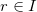(because I contains bq and c and is closed under subtraction and addition). However, recall that b was chosen to be the smallest positive element of I, so r must be equal to 0. Therefore c is a multiple of b, and we have shown that. Q.E.D.

Now back to Bézout's identity. We'll define: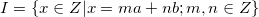This I is obviously non-empty and is closed under addition and subtraction (by its definition as a linear combination). Note, in particular, that it also contains a and b. By (III), I consists of all multiples of its smallest positive element, which we'll call d here.

To show that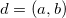we have to show that d|a, d|b and if c|a and c|b then c|d. First, by definition d is a divisor of any element in I, so it also divides a and b. If c|a and c|b, say a=cq and b=cp, then:So c|d, which completes our proof that d=(a,b). Q.E.D.

Regarding the corollary, it stems trivially from the definition of I and the proof above.